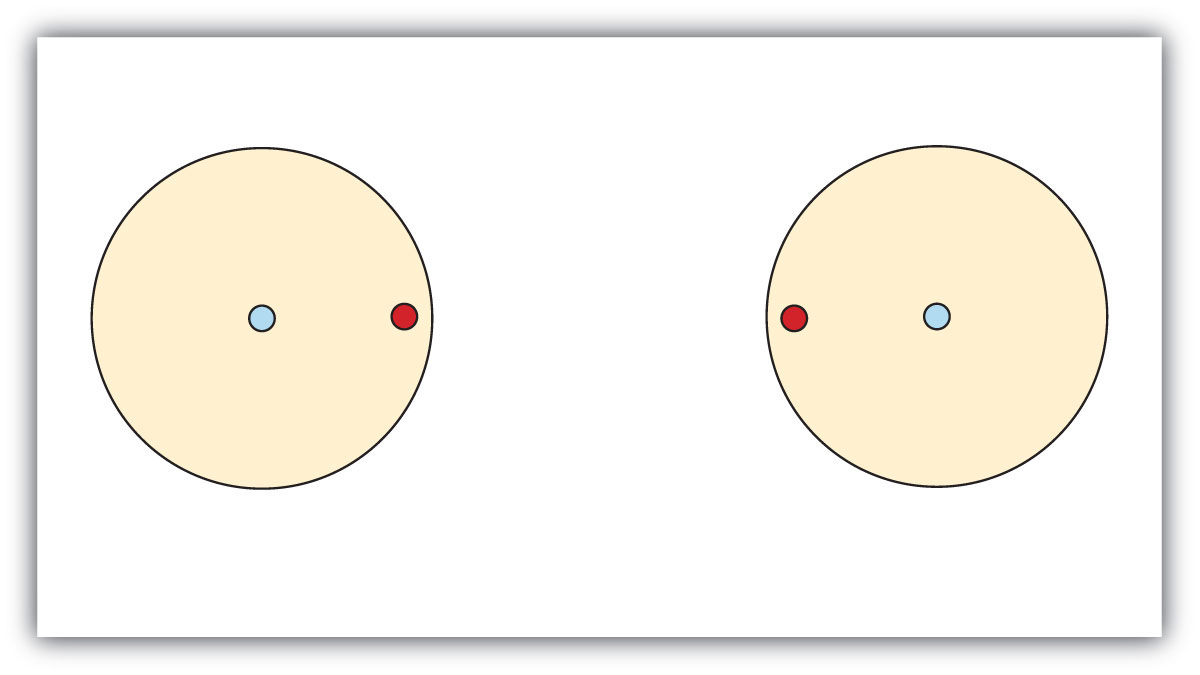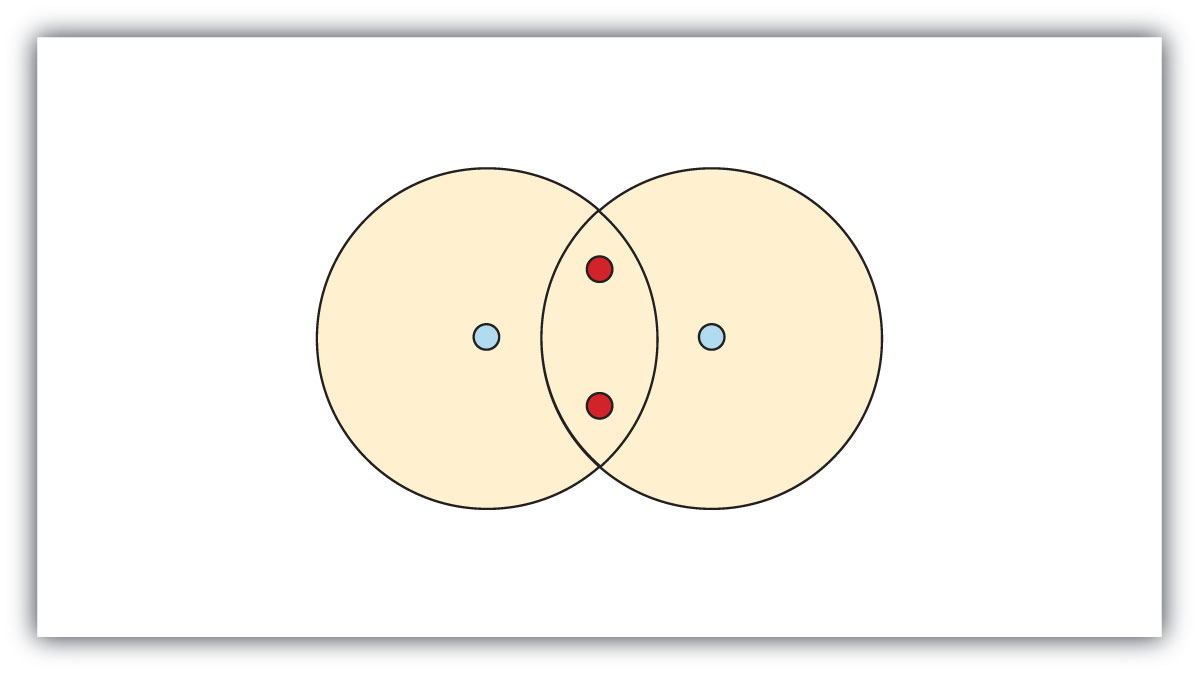## 4.1 Covalent Bonds

### Learning Objective

• Describe how a covalent bond forms.

You have already seen examples of substances that contain covalent bonds. One substance mentioned in Chapter 3 “Ionic Bonding and Simple Ionic Compounds” was water (H2O). You can tell from its formula that it is not an ionic compound; it is not composed of a metal and a nonmetal. Consequently, its properties are different from those of ionic compounds.

## Electron Sharing

Chapter 3 “Ionic Bonding and Simple Ionic Compounds” described how electrons can be transferred from one atom to another so that both atoms have an energy-stable outer electron shell. Because most filled electron shells have eight electrons in them, chemists called this tendency the octet rule. But there is another way an atom can achieve a full valence shell: atoms can share electrons.

This concept can be illustrated by using two hydrogen atoms, each of which has a single electron in its valence shell. (For small atoms such as hydrogen atoms, the valence shell will be the first shell, which holds only two electrons.) We can represent the two individual hydrogen atoms as follows:In contrast, when two hydrogen atoms get close enough together to share their electrons, they can be represented as follows:By sharing their valence electrons, both hydrogen atoms now have two electrons in their respective valence shells. Because each valence shell is now filled, this arrangement is more stable than when the two atoms are separate. The sharing of electrons between atoms is called a covalent bond, and the two electrons that join atoms in a covalent bond are called a bonding pair of electrons. A discrete group of atoms connected by covalent bonds is called a molecule—the smallest part of a compound that retains the chemical identity of that compound.

Chemists frequently use Lewis diagrams to represent covalent bonding in molecular substances. For example, the Lewis diagrams of two separate hydrogen atoms are as follows:The Lewis diagram of two hydrogen atoms sharing electrons looks like this:This depiction of molecules is simplified further by using a dash to represent a covalent bond. The hydrogen molecule is then represented as follows:Remember that the dash, also referred to as a single bond, represents a pair of electrons.

The bond in a hydrogen molecule, measured as the distance between the two nuclei, is about 7.4 × 10−11 m, or 74 picometers (pm; 1 pm = 1 × 10−12 m). This particular bond length represents a balance between several forces: the attractions between oppositely charged electrons and nuclei, the repulsion between two negatively charged electrons, and the repulsion between two positively charged nuclei. If the nuclei were closer together, they would repel each other more strongly; if the nuclei were farther apart, there would be less attraction between the positive and negative particles.

Fluorine is another element whose atoms bond together in pairs to form diatomic (two-atom) molecules. Two separate fluorine atoms have the following electron dot diagrams:Each fluorine atom contributes one valence electron, making a single bond and giving each atom a complete valence shell, which fulfills the octet rule:The circles show that each fluorine atom has eight electrons around it. As with hydrogen, we can represent the fluorine molecule with a dash in place of the bonding electrons:Each fluorine atom has six electrons, or three pairs of electrons, that are not participating in the covalent bond. Rather than being shared, they are considered to belong to a single atom. These are called nonbonding pairs (or lone pairs) of electrons.

## Covalent Bonds between Different Atoms

Now that we have looked at electron sharing between atoms of the same element, let us look at covalent bond formation between atoms of different elements. Consider a molecule composed of one hydrogen atom and one fluorine atom:Each atom needs one additional electron to complete its valence shell. By each contributing one electron, they make the following molecule:In this molecule, the hydrogen atom does not have nonbonding electrons, while the fluorine atom has six nonbonding electrons (three lone electron pairs). The circles show how the valence electron shells are filled for both atoms.

### Example 1

Draw the Lewis diagram for each compound.

1. a molecule composed of two chlorine atoms
2. a molecule composed of a hydrogen atom and a bromine atom

Solution

### Skill-Building Exercise

Draw the Lewis diagram for each compound.

1. a molecule composed of one chlorine atom and one fluorine atom

2. a molecule composed of one hydrogen atom and one iodine atom

Larger molecules are constructed in a similar fashion, with some atoms participating in more than one covalent bond. For example, water, with two hydrogen atoms and one oxygen atom, and methane (CH4), with one carbon atom and four hydrogen atoms, can be represented as follows:Atoms typically form a characteristic number of covalent bonds in compounds. Often the number of bonds is the same as the number of electrons short of an octet.

### Concept Review Exercises

1. How is a covalent bond formed between two atoms?
2. How does covalent bonding allow atoms in group 16 to satisfy the octet rule?

### Key Takeaway

• A covalent bond is formed between two atoms by sharing electrons.

### Exercises

1. Define covalent bond.

2. What is electron sharing?

3. Draw the Lewis diagram for the covalent bond in the Br2 molecule.

4. Draw the Lewis diagram for the covalent bond in the I2 molecule.

5. Draw the Lewis diagram for the covalent bond in the HCl molecule.

6. Draw the Lewis diagram for the covalent bond in the HI molecule.

7. What is the difference between a molecule and a formula unit?

8. Why do hydrogen atoms not follow the octet rule when they form covalent bonds?

9. Draw the Lewis diagram for the covalent bonding in H2S. How many bonding electrons and nonbonding electrons are in the molecule?

10. Draw the Lewis diagram for the covalent bonding in NI3. How many bonding electrons and nonbonding electrons are in the molecule?

11. Draw the Lewis diagram for the covalent bonding in CF4. How many bonding electrons and nonbonding electrons are in the molecule?

12. Draw the Lewis diagram for the covalent bonding in PCl3. How many bonding electrons and nonbonding electrons are in the molecule?

13. How many covalent bonds does a hydrogen atom typically form? Why?

14. How many covalent bonds does an oxygen atom typically form? Why?